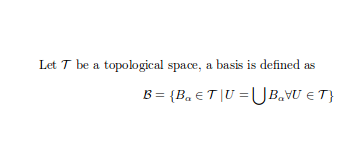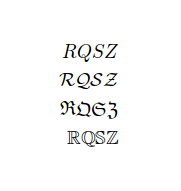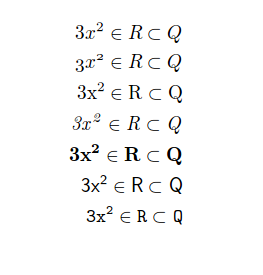Showing first {{hits.length}} results of {{hits_total}} for {{searchQueryText}}{{hits.length}} results for {{searchQueryText}}

Ничего не найдено

In mathematical mode as well as in text mode, you can change the typeface as needed. For instance, it's customary to represent real numbers with a blackboard bold font, or topological spaces with calligraphic font. This article shows several fonts for use in math mode. Contents 1 Introduction 2 Capital letters-only font typefaces 3 Other mathematical fonts 4 Further reading Introduction For some elements is convenient to have the possibility of changing the font typeface. Let $$\mathcal{T}$$ be a topological space, a basis is defined as $\mathcal{B} = \{B_{\alpha} \in \mathcal{T}\, |\, U = \bigcup B_{\alpha} \forall U \in \mathcal{T} \}$Several fonts require the addition of the line \usepackage{amssymb} to the preamble to work.   Open an example in Overleaf Capital letters-only font typefaces There are some font typefaces that support only a limited number of characters; these fonts usually denote some special sets. \begin{align*} RQSZ \\ \mathcal{RQSZ} \\ \mathfrak{RQSZ} \\ \mathbb{RQSZ} \end{align*}This example shows Calligraphic, Fraktur and Blackboard bold typefaces. For instance, to display the R in blackboard bold typeface $\mathbb{R}$ will do the trick.   Open an example in Overleaf Other mathematical fonts It's possible to set a different font family for a complete mathematical expression. \begin{align*} 3x^2 \in R \subset Q \\ \mathnormal{3x^2 \in R \subset Q} \\ \mathrm{3x^2 \in R \subset Q} \\ \mathit{3x^2 \in R \subset Q} \\ \mathbf{3x^2 \in R \subset Q} \\ \mathsf{3x^2 \in R \subset Q} \\ \mathtt{3x^2 \in R \subset Q} \end{align*}In this case, not only letters but all characters change its appearance, for example $\mathit{3x^2}$ italicises the entire expression.   Open an example in Overleaf Further reading For more information see Mathematical expressions Subscripts and superscripts Bold, italics and underlining Font sizes, families, and styles Font typefaces Text alignment The not so short introduction to LaTeX2ε